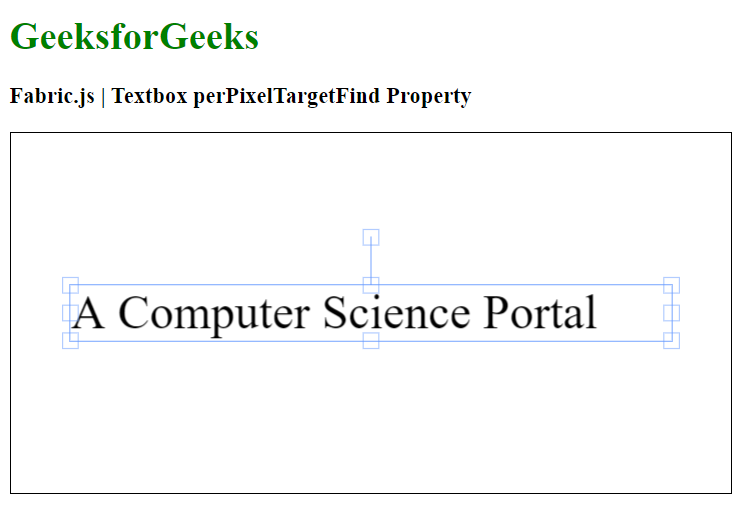Related Articles

# Fabric.js Textbox perPixelTargetFind Property

• Last Updated : 20 Jan, 2021

In this article, we are going to see how to set the value of perPixelTargetFind property of a canvas textbox using FabricJS. The canvas textbox means textbox is movable and can be stretched according to requirement. Further, the textbox can be customized when it comes to initial stroke color, height, width, fill color, or stroke width.

To make it possible, we are going to use a JavaScript library called FabricJS. After importing the library, we will create a canvas block in the body tag which will contain the textbox. After this, we will initialize instances of Canvas and textbox provided by FabricJS and set the perPixelTargetFind property value of the textbox using perPixelTargetFind property and render the textbox on the Canvas as given in the example below.

Syntax:

```fabric.Textbox('text', {
perPixelTargetFind: boolean
});```

Parameters: This function accepts three parameters as mentioned above and described below:

• perPixelTargetFind: It specifies the string value of perPixelTargetFind property.

Example: This example uses FabricJS to set the perPixelTargetFind property of a canvas textbox.

## HTML

 ```<``html``>`` ` `<``head``>``    ````    ``<``script` `src``=``"https://cdnjs.cloudflare.com/ajax/libs/fabric.js/3.6.2/fabric.min.js"``>``    `````` ` `<``body``>``    ``<``h1` `style``=``"color: green;"``>``        ``GeeksforGeeks``    ```` ` `    ``<``h3``>``        ``Fabric.js | Textbox perPixelTargetFind Property``    ```` ` `    ``<``canvas` `id``=``"canvas"` `width``=``"600"` `height``=``"300"``        ``style``=``"border:1px solid #000000"``>``    ```` ` `    ``<``script``>``        ``// Initiate a Canvas instance ``        ``var canvas = new fabric.Canvas("canvas");`` ` `        ``// Create a new Textbox instance ``        ``var text = new fabric.Textbox(``            ``'A Computer Science Portal', {``            ``width: 500,``            ``perPixelTargetFind: true``        ``});`` ` `        ``// Render the Textbox in canvas ``        ``canvas.add(text);``        ``canvas.centerObject(text);``    `````` ` ``

Output:My Personal Notes arrow_drop_up